# Chapter 8 Chemical Equations Reactions 8 1 Reactions

• Slides: 19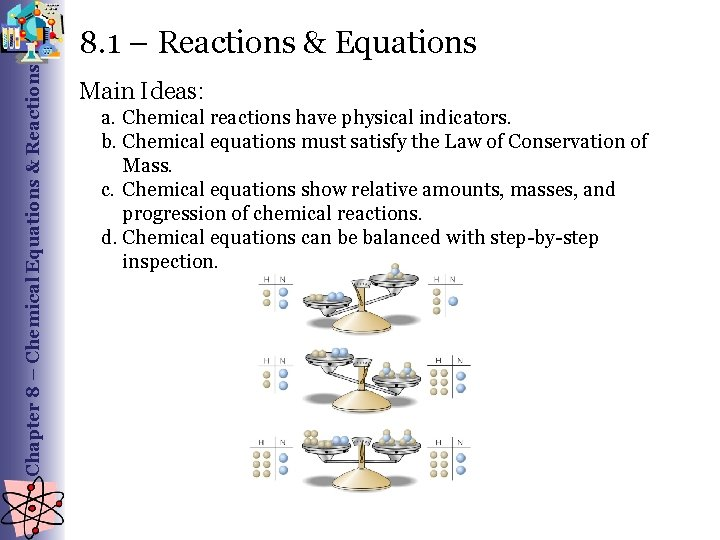Chapter 8 – Chemical Equations & Reactions 8. 1 – Reactions & Equations Main Ideas: a. Chemical reactions have physical indicators. b. Chemical equations must satisfy the Law of Conservation of Mass. c. Chemical equations show relative amounts, masses, and progression of chemical reactions. d. Chemical equations can be balanced with step-by-step inspection.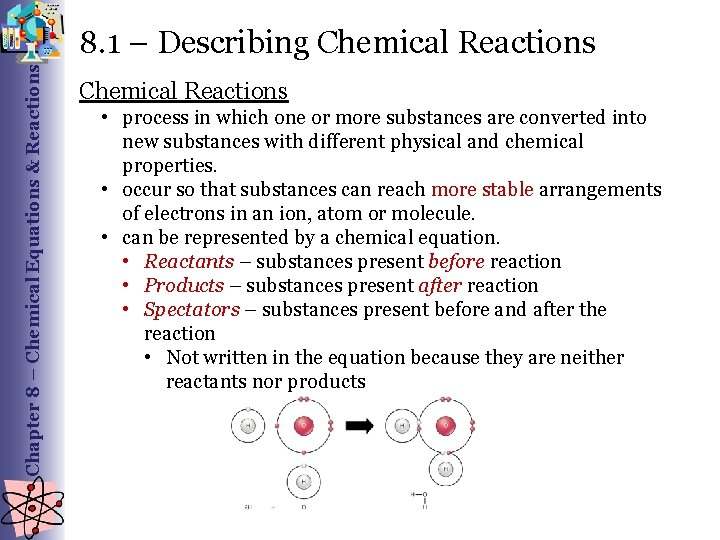Chapter 8 – Chemical Equations & Reactions 8. 1 – Describing Chemical Reactions • process in which one or more substances are converted into new substances with different physical and chemical properties. • occur so that substances can reach more stable arrangements of electrons in an ion, atom or molecule. • can be represented by a chemical equation. • Reactants – substances present before reaction • Products – substances present after reaction • Spectators – substances present before and after the reaction • Not written in the equation because they are neither reactants nor products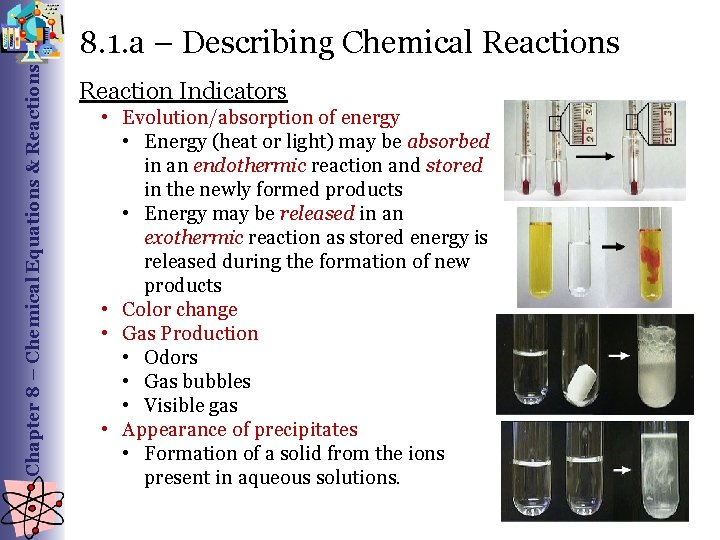Chapter 8 – Chemical Equations & Reactions 8. 1. a – Describing Chemical Reactions Reaction Indicators • Evolution/absorption of energy • Energy (heat or light) may be absorbed in an endothermic reaction and stored in the newly formed products • Energy may be released in an exothermic reaction as stored energy is released during the formation of new products • Color change • Gas Production • Odors • Gas bubbles • Visible gas • Appearance of precipitates • Formation of a solid from the ions present in aqueous solutions.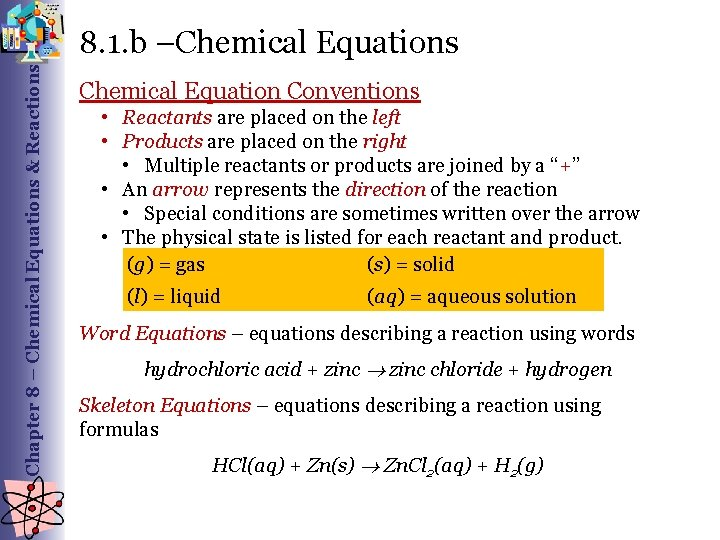Chapter 8 – Chemical Equations & Reactions 8. 1. b –Chemical Equations Chemical Equation Conventions • Reactants are placed on the left • Products are placed on the right • Multiple reactants or products are joined by a “+” • An arrow represents the direction of the reaction • Special conditions are sometimes written over the arrow • The physical state is listed for each reactant and product. (g) = gas (s) = solid (l) = liquid (aq) = aqueous solution Word Equations – equations describing a reaction using words hydrochloric acid + zinc chloride + hydrogen Skeleton Equations – equations describing a reaction using formulas HCl(aq) + Zn(s) Zn. Cl 2(aq) + H 2(g)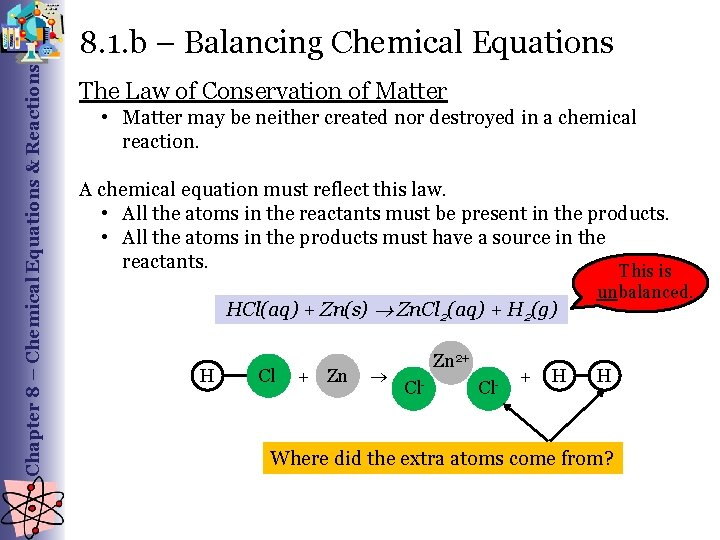Chapter 8 – Chemical Equations & Reactions 8. 1. b – Balancing Chemical Equations The Law of Conservation of Matter • Matter may be neither created nor destroyed in a chemical reaction. A chemical equation must reflect this law. • All the atoms in the reactants must be present in the products. • All the atoms in the products must have a source in the reactants. This is unbalanced. HCl(aq) + Zn(s) Zn. Cl 2(aq) + H 2(g) H Cl + Zn Zn 2+ Cl- + H H Where did the extra atoms come from?Chapter 8 – Chemical Equations & Reactions 8. 1. d – Balancing Chemical Equations Balancing an equation is really about finding ratios that occur in reactions that satisfy the Law of Conservation of Matter. • A single set of ratios will exist for every chemical reaction • Coefficients are numbers used to show many molecules, ions or atoms are present in a reaction. This is balanced. 2 HCl(aq) + Zn(s) Zn. Cl 2(aq) + H 2(g) “ 2” is a coefficient. H Cl + Zn Zn 2+ Cl- + H Adding a second HCl provides the atoms we need to have a balanced equation. H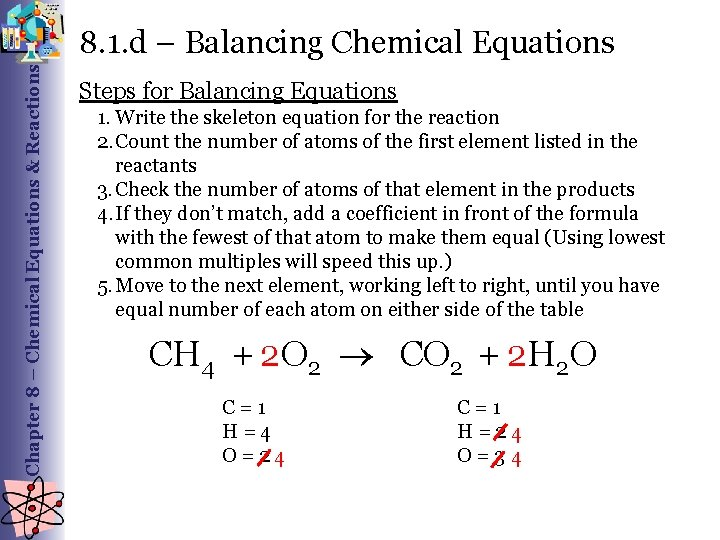Chapter 8 – Chemical Equations & Reactions 8. 1. d – Balancing Chemical Equations Steps for Balancing Equations 1. Write the skeleton equation for the reaction 2. Count the number of atoms of the first element listed in the reactants 3. Check the number of atoms of that element in the products 4. If they don’t match, add a coefficient in front of the formula with the fewest of that atom to make them equal (Using lowest common multiples will speed this up. ) 5. Move to the next element, working left to right, until you have equal number of each atom on either side of the table 2 2 O CH 4 + O 2 2 CO 2 + H C = 1 H = 4 O = 2 4 C = 1 H = 2 4 O = 3 4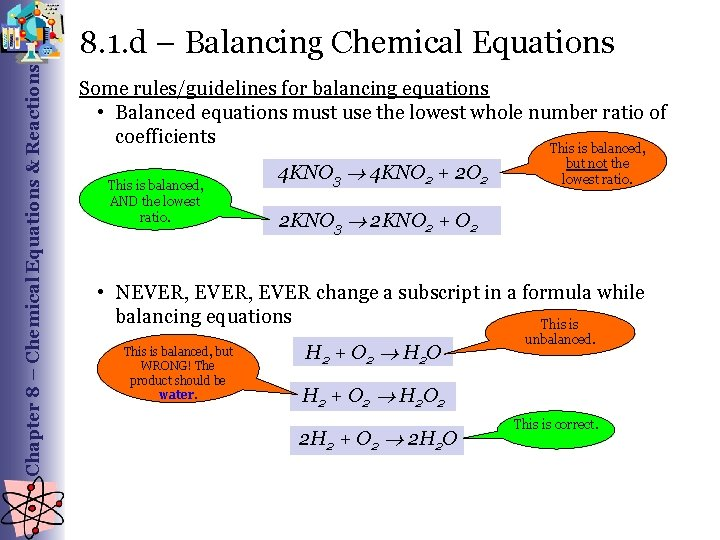Chapter 8 – Chemical Equations & Reactions 8. 1. d – Balancing Chemical Equations Some rules/guidelines for balancing equations • Balanced equations must use the lowest whole number ratio of coefficients This is balanced, AND the lowest ratio. 4 KNO 3 4 KNO 2 + 2 O 2 but not the lowest ratio. 2 KNO 3 2 KNO 2 + O 2 • NEVER, EVER change a subscript in a formula while balancing equations This is balanced, but WRONG! The product should be water. H 2 + O 2 H 2 O unbalanced. H 2 + O 2 H 2 O 2 2 H 2 + O 2 2 H 2 O This is correct.Chapter 8 – Chemical Equations & Reactions 8. 1. d – Balancing Chemical Equations • Balance single species substances last. C 3 H 8 + O 2 CO 2 + H 2 O If I change the coefficient here, I only affect O. If I change the coefficient here, I affect O and C.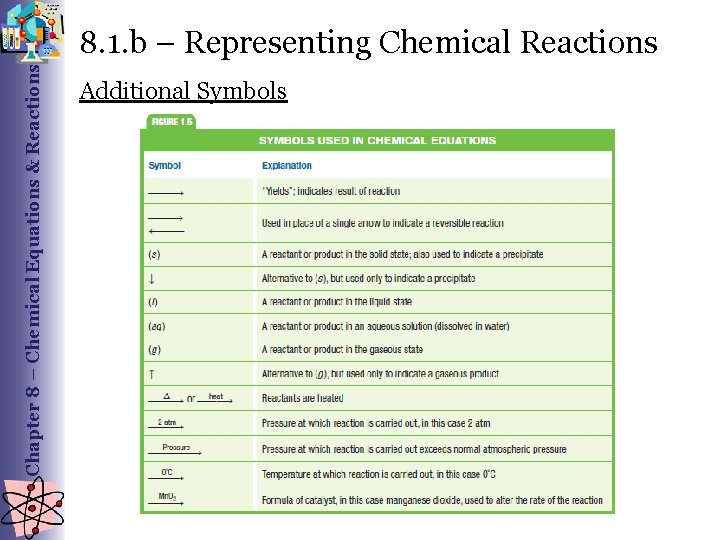Chapter 8 – Chemical Equations & Reactions 8. 1. b – Representing Chemical Reactions Additional Symbols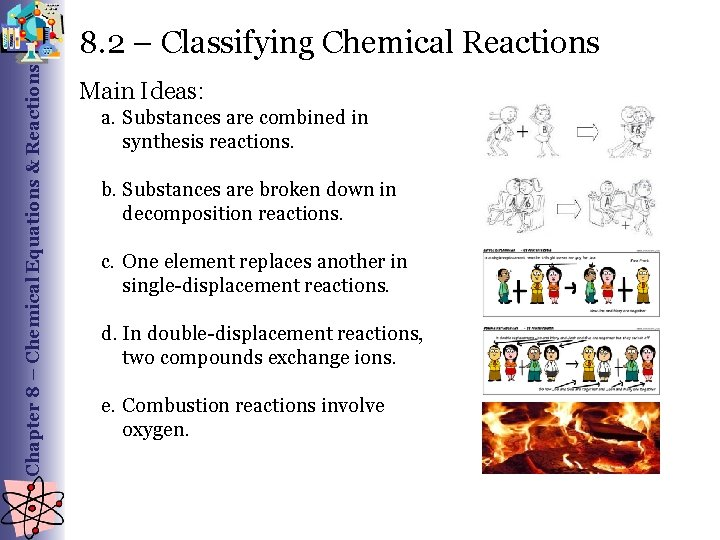Chapter 8 – Chemical Equations & Reactions 8. 2 – Classifying Chemical Reactions Main Ideas: a. Substances are combined in synthesis reactions. b. Substances are broken down in decomposition reactions. c. One element replaces another in single-displacement reactions. d. In double-displacement reactions, two compounds exchange ions. e. Combustion reactions involve oxygen.Chapter 8 – Chemical Equations & Reactions 8. 2. a – Classifying Chemical Reactions Synthesis Reactions • Two or more substances combine to form one new substance. NH 3(aq) + HCl(aq) NH 4 Cl(g) 2 H 2(g) + O 2(g) 2 H 2 O(l) Synthesis reactions may be recognized by the general form of the equation: Substance + Substance Compound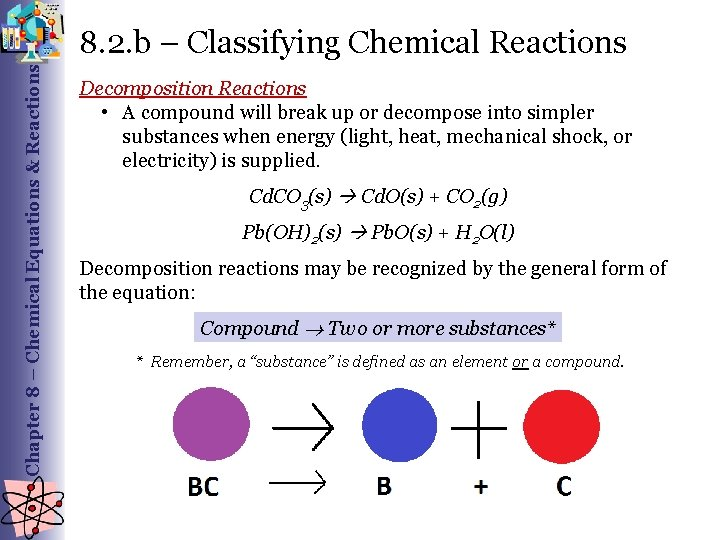Chapter 8 – Chemical Equations & Reactions 8. 2. b – Classifying Chemical Reactions Decomposition Reactions • A compound will break up or decompose into simpler substances when energy (light, heat, mechanical shock, or electricity) is supplied. CO 3(s) Cd. O(s) + CO 2(g) Pb(OH)2(s) Pb. O(s) + H 2 O(l) Decomposition reactions may be recognized by the general form of the equation: Compound Two or more substances* * Remember, a “substance” is defined as an element or a compound.Chapter 8 – Chemical Equations & Reactions 8. 2. c – Classifying Chemical Reactions Single Replacement Reactions • One element replaces another in a compound. Cl 2(g) + 2 KBr(aq) 2 KCl(aq) + Br 2(l) 2 Al(s) + Fe 2 O 3(aq) 2 Fe(s) + Al 2 O 3(aq) Single replacement reactions may be recognized by the general form of the equation: Element + CompoundChapter 8 – Chemical Equations & Reactions 8. 2. d – Classifying Chemical Reactions Double Replacement Reactions • The positive and negative portions of two compounds are interchanged. Ag. NO 3(aq) + Na. OH(aq) Ag. OH(s) + Na. NO 3(aq) 3 Ni. SO 4(aq) + 2 Li 3 PO 4(aq) Ni 3(PO 4)2(s) + 3 Li 2 SO 4(aq) Double replacement reactions may be recognized by the general form of the equation: Compound + Compound • Solids formed in an aqueous double replacement reaction are called precipitates. • Products will include a precipitate, a gas or water. + AB 2 + CD + AD + CB 2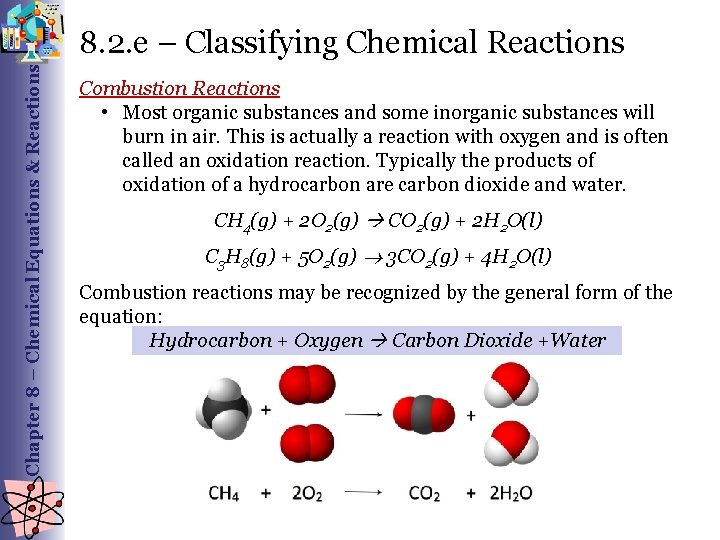Chapter 8 – Chemical Equations & Reactions 8. 2. e – Classifying Chemical Reactions Combustion Reactions • Most organic substances and some inorganic substances will burn in air. This is actually a reaction with oxygen and is often called an oxidation reaction. Typically the products of oxidation of a hydrocarbon are carbon dioxide and water. CH 4(g) + 2 O 2(g) CO 2(g) + 2 H 2 O(l) C 3 H 8(g) + 5 O 2(g) 3 CO 2(g) + 4 H 2 O(l) Combustion reactions may be recognized by the general form of the equation: Hydrocarbon + Oxygen Carbon Dioxide +WaterChapter 8 – Chemical Equations & Reactions 8. 3 – Activity Series of Elements Main Ideas: 1. An activity series helps determine what substances will displace others in chemical reactions.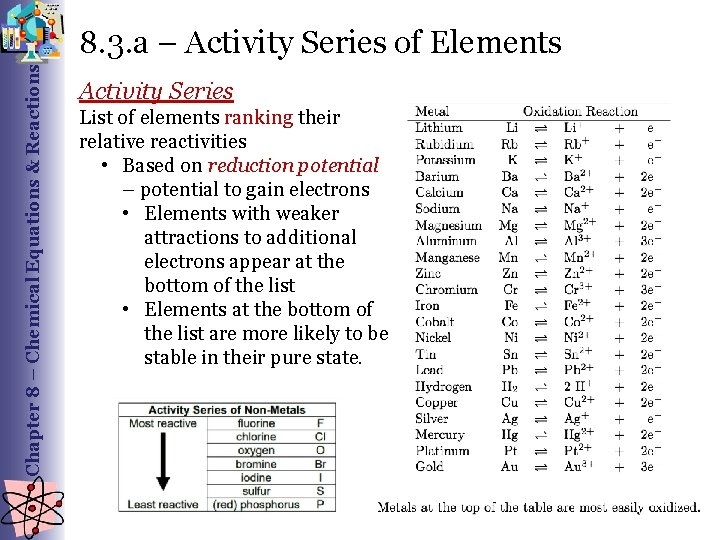Chapter 8 – Chemical Equations & Reactions 8. 3. a – Activity Series of Elements Activity Series List of elements ranking their relative reactivities • Based on reduction potential – potential to gain electrons • Elements with weaker attractions to additional electrons appear at the bottom of the list • Elements at the bottom of the list are more likely to be stable in their pure state.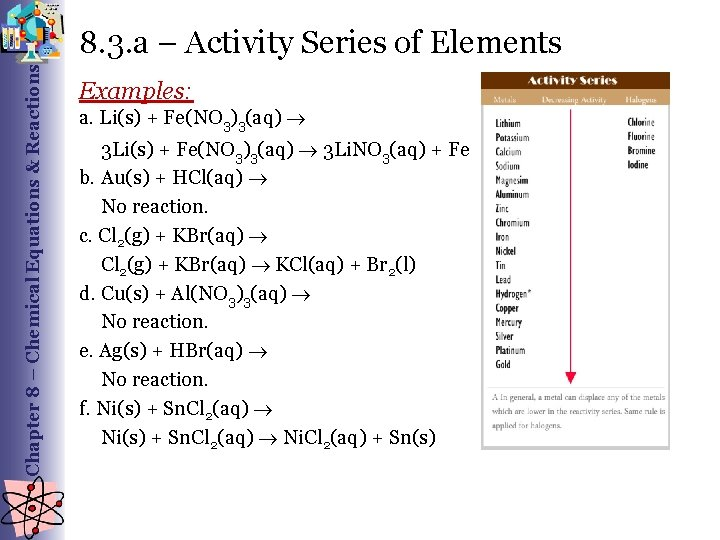Chapter 8 – Chemical Equations & Reactions 8. 3. a – Activity Series of Elements Examples: a. Li(s) + Fe(NO 3)3(aq) 3 Li. NO 3(aq) + Fe b. Au(s) + HCl(aq) No reaction. c. Cl 2(g) + KBr(aq) KCl(aq) + Br 2(l) d. Cu(s) + Al(NO 3)3(aq) No reaction. e. Ag(s) + HBr(aq) No reaction. f. Ni(s) + Sn. Cl 2(aq) Ni. Cl 2(aq) + Sn(s)